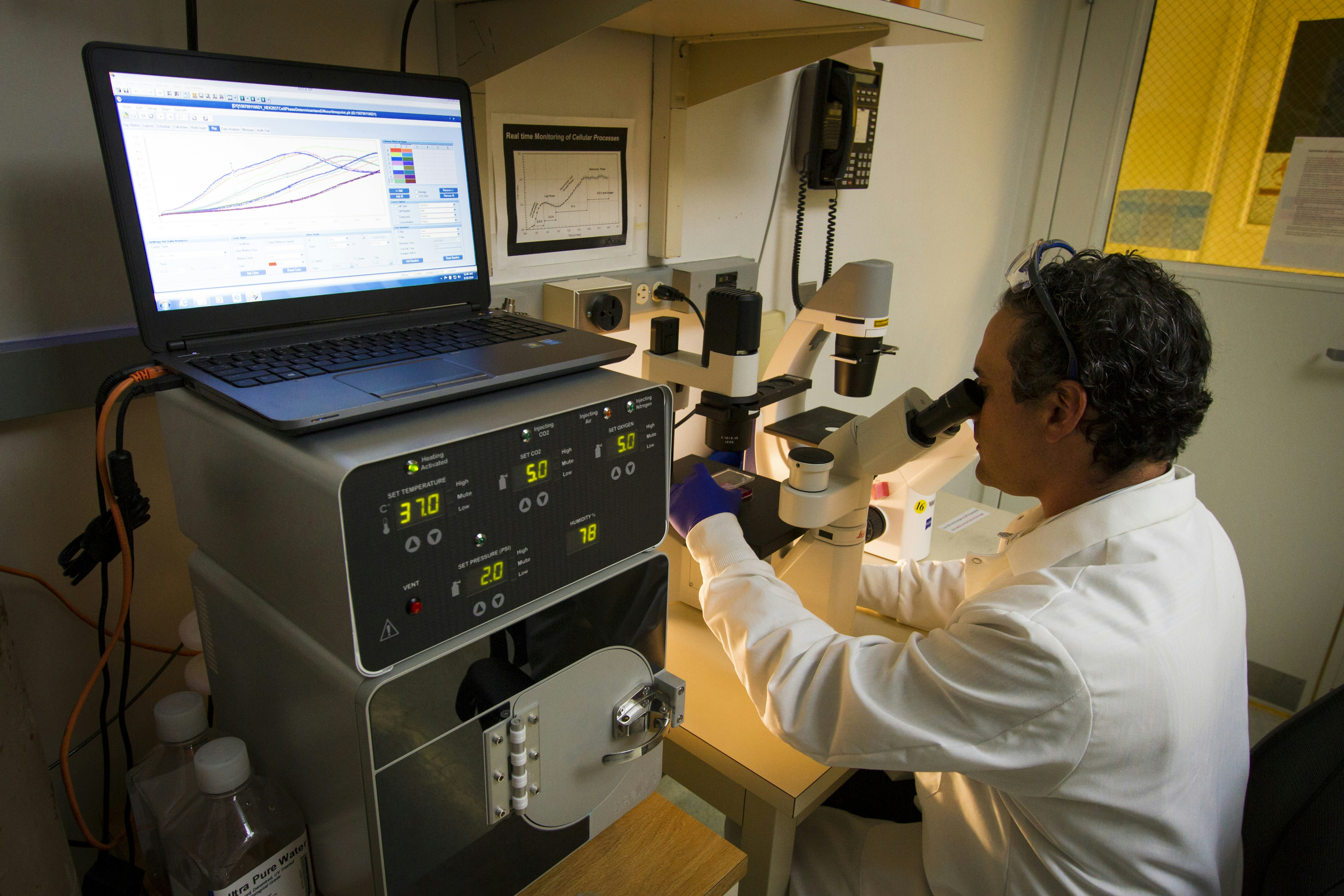Journal article //

Statistical Analysis of Random Walks on Network
2021
A. Kalikova

### Metrics

•0 views
•0 downloads0 views  //  0 downloadsAbstract

This paper describes an investigation of analytical formulas for parameters in random walks. Random walks are used to model situations in which an object moves in a sequence of steps in randomly chosen directions. Given a graph and a starting point, we select a neighbor of it at random, and move to this neighbor; then we select a neighbor of this point at random, and move to it etc. It is a fundamental dynamic process that arises in many models in mathematics, physics, informatics and can be used to model random processes inherent to many important applications. Different aspects of the theory of random walks on graphs are surveyed. In particular, estimates on the important parameters of hitting time, commute time, cover time are discussed in various works. In some papers, authors have derived an analytical expression for the distribution of the cover time for a random walk over an arbitrary graph that was tested for small values of n. However, this work will show the simplified analytical expressions for distribution of hitting time, commute time, cover time for bigger values of n. Moreover, this work will present the probability mass function and the cumulative distribution function for hitting time, commute time.

Full text

### Metrics

•0 views
•0 downloads0 views  //  0 downloads